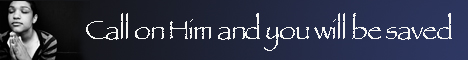Note:  Do not rely on this information. It is very old.

# Square Root

Square-Root. If a2 = b, then a is said to be the square-root of b, where b is any expression. For real expressions the method of finding the square-root is a modification of the process of long division, the method used in arithmetic being simply deduced from algebra. If we are dealing with a perfect square, it is of the form x2 + 2 x y + y2, or x2 and y (2 x + y), and this forms the basis of the rule by which x and y would be found in turn. The square-roots of expressions containing surds and imaginary quantities are found by special methods. A practical method of finding the square-root of a number is to use logarithms. For since a2 = b, log a2 = log b, ... 2 log a = loq b; hence the rule is to look up the logarithm of the number and halve it. The result is the logarithm of the required root.